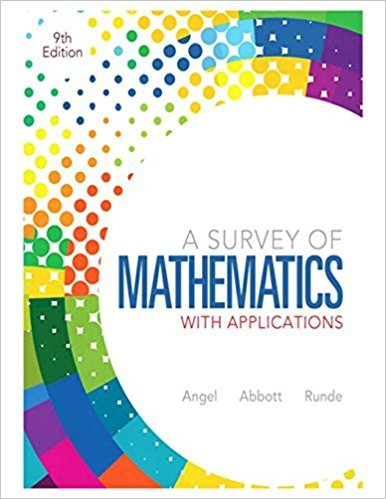×
×

# Solutions for Chapter 11: Consumer Mathematics## Full solutions for A Survey of Mathematics with Applications | 9th Edition

ISBN: 9780321759665Solutions for Chapter 11: Consumer Mathematics

Solutions for Chapter 11
4 5 0 393 Reviews
13
1
##### ISBN: 9780321759665

Chapter 11: Consumer Mathematics includes 66 full step-by-step solutions. This expansive textbook survival guide covers the following chapters and their solutions. This textbook survival guide was created for the textbook: A Survey of Mathematics with Applications, edition: 9. A Survey of Mathematics with Applications was written by and is associated to the ISBN: 9780321759665. Since 66 problems in chapter 11: Consumer Mathematics have been answered, more than 80696 students have viewed full step-by-step solutions from this chapter.

Key Math Terms and definitions covered in this textbook
• Cayley-Hamilton Theorem.

peA) = det(A - AI) has peA) = zero matrix.

• Condition number

cond(A) = c(A) = IIAIlIIA-III = amaxlamin. In Ax = b, the relative change Ilox III Ilx II is less than cond(A) times the relative change Ilob III lib II· Condition numbers measure the sensitivity of the output to change in the input.

• Diagonalizable matrix A.

Must have n independent eigenvectors (in the columns of S; automatic with n different eigenvalues). Then S-I AS = A = eigenvalue matrix.

• Dimension of vector space

dim(V) = number of vectors in any basis for V.

• Distributive Law

A(B + C) = AB + AC. Add then multiply, or mUltiply then add.

• Echelon matrix U.

The first nonzero entry (the pivot) in each row comes in a later column than the pivot in the previous row. All zero rows come last.

• Ellipse (or ellipsoid) x T Ax = 1.

A must be positive definite; the axes of the ellipse are eigenvectors of A, with lengths 1/.JI. (For IIx II = 1 the vectors y = Ax lie on the ellipse IIA-1 yll2 = Y T(AAT)-1 Y = 1 displayed by eigshow; axis lengths ad

• Hessenberg matrix H.

Triangular matrix with one extra nonzero adjacent diagonal.

• Iterative method.

A sequence of steps intended to approach the desired solution.

• Kronecker product (tensor product) A ® B.

Blocks aij B, eigenvalues Ap(A)Aq(B).

• Length II x II.

Square root of x T x (Pythagoras in n dimensions).

• Partial pivoting.

In each column, choose the largest available pivot to control roundoff; all multipliers have leij I < 1. See condition number.

• Pivot columns of A.

Columns that contain pivots after row reduction. These are not combinations of earlier columns. The pivot columns are a basis for the column space.

• Pivot.

The diagonal entry (first nonzero) at the time when a row is used in elimination.

• Plane (or hyperplane) in Rn.

Vectors x with aT x = O. Plane is perpendicular to a =1= O.

• Rank one matrix A = uvT f=. O.

Column and row spaces = lines cu and cv.

• Reduced row echelon form R = rref(A).

Pivots = 1; zeros above and below pivots; the r nonzero rows of R give a basis for the row space of A.

• Special solutions to As = O.

One free variable is Si = 1, other free variables = o.

• Triangle inequality II u + v II < II u II + II v II.

For matrix norms II A + B II < II A II + II B II·

• Vandermonde matrix V.

V c = b gives coefficients of p(x) = Co + ... + Cn_IXn- 1 with P(Xi) = bi. Vij = (Xi)j-I and det V = product of (Xk - Xi) for k > i.

×